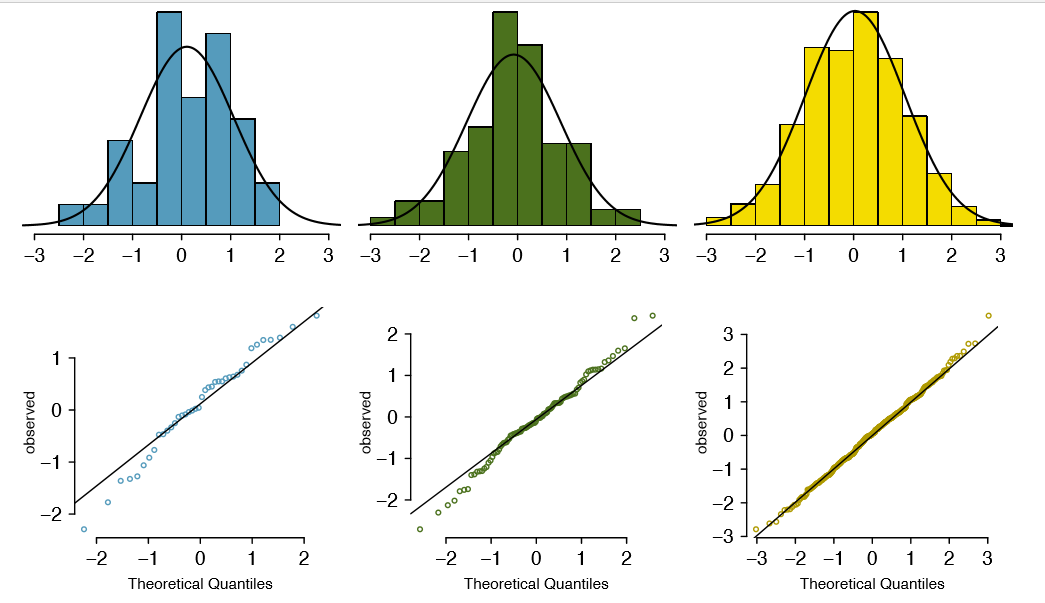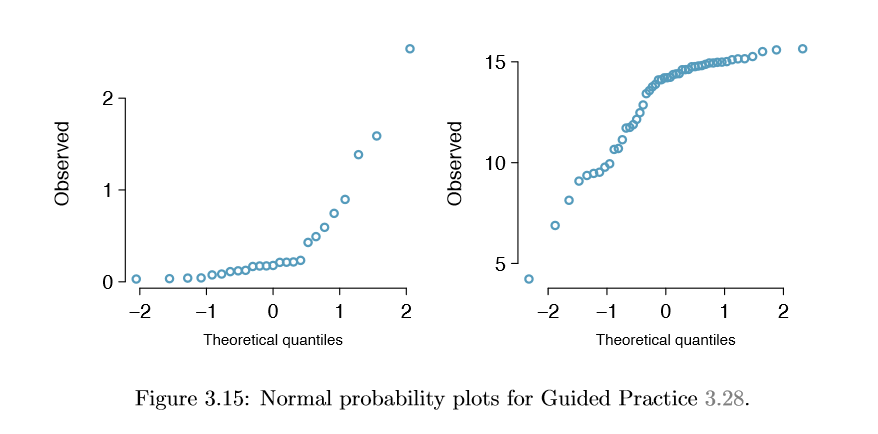# 3.2: Evaluating the Normal Approximation

Many processes can be well approximated by the normal distribution. We have already seen two good examples: SAT scores and the heights of US adult males. While using a normal model can be extremely convenient and helpful, it is important to remember normality is always an approximation. Testing the appropriateness of the normal assumption is a key step in many data analyses.Figure 3.10: A sample of 100 male heights. The observations are rounded to the nearest whole inch, explaining why the points appear to jump in increments in the normal probability plot.Normal probability plot

Example 3.15 suggests the distribution of heights of US males is well approximated by the normal model. We are interested in proceeding under the assumption that the data are normally distributed, but first we must check to see if this is reasonable. There are two visual methods for checking the assumption of normality, which can be implemented and interpreted quickly. The first is a simple histogram with the best tting normal curve overlaid on the plot, as shown in the left panel of Figure 3.10. The sample mean $$\bar {x}$$ and standard deviation s are used as the parameters of the best tting normal curve. The closer this curve ts the histogram, the more reasonable the normal model assumption. Another more common method is examining a normal probability plot.19, shown in the right panel of Figure 3.10. The closer the points are to a perfect straight line, the more con dent we can be that the data follow the normal model. We outline the construction of the normal probability plot in Section 3.2.2

Example 3.24

Three data sets of 40, 100, and 400 samples were simulated from a normal distribution, and the histograms and normal probability plots of the data sets are shown in Figure 3.11. These will provide a benchmark for what to look for in plots of real data.

The left panels show the histogram (top) and normal probability plot (bottom) for the simulated data set with 40 observations. The data set is too small to really see clear structure in the histogram. The normal probability plot also reects this, where there are some deviations from the line. However, these deviations are not strong. The middle panels show diagnostic plots for the data set with 100 simulated observations. The histogram shows more normality and the normal probability plot shows a better fit. While there is one observation that deviates noticeably from the line, it is not particularly extreme.

19Also commonly called a quantile-quantile plot.Figure 3.11: Histograms and normal probability plots for three simulated normal data sets; n = 40 (left), n = 100 (middle), n = 400 (right).

The data set with 400 observations has a histogram that greatly resembles the normal distribution, while the normal probability plot is nearly a perfect straight line. Again in the normal probability plot there is one observation (the largest) that deviates slightly from the line. If that observation had deviated 3 times further from the line, it would be of much greater concern in a real data set. Apparent outliers can occur in normally distributed data but they are rare.

Notice the histograms look more normal as the sample size increases, and the normal probability plot becomes straighter and more stable.

Example

Example 3.25 Are NBA player heights normally distributed? Consider all 435 NBA players from the 2008-9 season presented in Figure 3.12.20

We first create a histogram and normal probability plot of the NBA player heights. The histogram in the left panel is slightly left skewed, which contrasts with the symmetric normal distribution. The points in the normal probability plot do not appear to closely follow a straight line but show what appears to be a \wave". We can compare these characteristics to the sample of 400 normally distributed observations in Example 3.24 and see that they represent much stronger deviations from the normal model. NBA player heights do not appear to come from a normal distribution.

20These data were collected from http://www.nba.com.Figure 3.12: Histogram and normal probability plot for the NBA heights from the 2008-9 season.

Example

Example 3.26 Can we approximate poker winnings by a normal distribution? We consider the poker winnings of an individual over 50 days. A histogram and normal probability plot of these data are shown in Figure 3.13.Figure 3.13: A histogram of poker data with the best fitting normal plot and a normal probability plot.

The data are very strongly right skewed in the histogram, which corresponds to the very strong deviations on the upper right component of the normal probability plot. If we compare these results to the sample of 40 normal observations in Example 3.24, it is apparent that these data show very strong deviations from the normal model.

Exercise 3.27

Determine which data sets represented in Figure 3.14 plausibly come from a nearly normal distribution. Are you con dent in all of your conclusions? There are 100 (top left), 50 (top right), 500 (bottom left), and 15 points (bottom right) in the four plots.21Figure 3.14: Four normal probability plots for Exercise 3.27.

Exercise 3.28

Figure 3.15 shows normal probability plots for two distributions that are skewed. One distribution is skewed to the low end (left skewed) and the other to the high end (right skewed). Which is which?22Figure 3.15: Normal probability plots for Exercise 3.28.

21Answers may vary a little. The top-left plot shows some deviations in the smallest values in the data set; speci cally, the left tail of the data set has some outliers we should be wary of. The top-right and bottom-left plots do not show any obvious or extreme deviations from the lines for their respective sample sizes, so a normal model would be reasonable for these data sets. The bottom-right plot has a consistent curvature that suggests it is not from the normal distribution. If we examine just the vertical coordinates of these observations, we see that there is a lot of data between -20 and 0, and then about ve observations scattered between 0 and 70. This describes a distribution that has a strong right skew.

22Examine where the points fall along the vertical axis. In the first plot, most points are near the low end with fewer observations scattered along the high end; this describes a distribution that is skewed to the high end. The second plot shows the opposite features, and this distribution is skewed to the low end.Constructing a normal probability plot (special topic)

We construct a normal probability plot for the heights of a sample of 100 men as follows:

1. Order the observations.
2. Determine the percentile of each observation in the ordered data set.
3. Identify the Z score corresponding to each percentile.
4. Create a scatterplot of the observations (vertical) against the Z scores (horizontal).

If the observations are normally distributed, then their Z scores will approximately correspond to their percentiles and thus to the $$z_i$$ in Table 3.16.

Table 3.16: Construction details for a normal probability plot of 100 men's heights. The first observation is assumed to be at the 0:99th percentile, and the $$z_i$$ corresponding to a lower tail of 0.0099 is -2:33. To create the plot based on this table, plot each pair of points, ($$z_i$$, $$x_i$$).
Observation i 1 2 3 $$\dots$$ 100

$$x_i$$

61

63

63

$$\dots$$

78

Percentile 0.99% 1.98% 2.97% $$\dots$$ 99.01%
$$z_i$$ -2.33 -2.06 -1.89 $$\dots$$ 2.33

Caution: $$z_i$$ correspond to percentiles

The $$z_i$$ in Table 3.16 are not the Z scores of the observations but only correspond to the percentiles of the observations.

Because of the complexity of these calculations, normal probability plots are generally created using statistical software.

## Contributors

• David M Diez (Google/YouTube), Christopher D Barr (Harvard School of Public Health), Mine Çetinkaya-Rundel (Duke University)